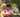### MaxDoubleSliceSum

February 12, 2020

MaxDoubleSliceSum

A non-empty array A consisting of N integers is given. A triplet (X, Y, Z), such that 0 ≤ X < Y < Z < N, is called a double slice. The sum of double slice (X, Y, Z) is the total of A[X + 1] + A[X + 2] + ... + A[Y − 1] + A[Y + 1] + A[Y + 2] + ... + A[Z − 1].
For example, array A such that:
A = 3
A = 2
A = 6
A = -1
A = 4
A = 5
A = -1
A = 2
contains the following example double slices:
• double slice (0, 3, 6), sum is 2 + 6 + 4 + 5 = 17,
• double slice (0, 3, 7), sum is 2 + 6 + 4 + 5 − 1 = 16,
• double slice (3, 4, 5), sum is 0.
The goal is to find the maximal sum of any double slice.

Write a function:
function solution(A);
that, given a non-empty array A consisting of N integers, returns the maximal sum of any double slice.
For example, given:
A = 3
A = 2
A = 6
A = -1
A = 4
A = 5
A = -1
A = 2
the function should return 17, because no double slice of array A has a sum of greater than 17.
Write an efficient algorithm for the following assumptions:
• N is an integer within the range [3..100,000];
• each element of array A is an integer within the range [−10,000..10,000].

``````function solution(A) {
// write your code in JavaScript (Node.js 8.9.4)
if(A.length<4) return 0
let L = [], sumL = 0
let R = [], sumR = 0
let max = A
let len = A.length
let i
L=0 //first
for(i=2; i<len-1; i++){
sumL += A[i-1]
if(sumL<0) sumL = 0
L[i-1] = sumL
}
R[len-1]=0 /last
for(i=len-3; i>=1; i--){
sumR += A[i+1]
if(sumR<0) sumR = 0
R[i+1] = sumR
}

for(i=1; i<len-1; i++){ // last i=len-2
if(L[i-1]+R[i+1]>max) max = L[i-1]+R[i+1]
}
// L+R; L+R; ---- L[len-3]+R[len-1]

return max
}``````Posted by Tai Lu ( 呂台生 ) who's building useful things.You should follow him on Twitter